# ISEE Upper Level Quantitative : How to find the surface area of a pyramid

## Example Questions

### Example Question #1 : How To Find The Surface Area Of A Pyramid

A pyramid with a square base has height equal to the perimeter of its base. Which is the greater quantity?

(A) Twice the area of its base

(B) The area of one of its triangular faces

(A) is greater

(A) and (B) are equal

(B) is greater

It is impossible to determine which is greater from the information given

(B) is greater

Explanation:

Since the answer is not dependent on the actual dimensions, for the sake of simplicity, we assume that the base has sidelength 2. Then the area of the base is the square of this, or 4.

The height of the pyramid is equal to the perimeter of the base, or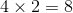. A right triangle can be formed with the lengths of its legs equal to the height of the pyramid, or 8, and one half the length of a side, or 1; the length of its hypotenuse, which is the slant height, is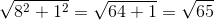This is the height of one triangular face; its base is a side of the square, so the length of the base is 2. The area of a face is half the product of these dimensions, or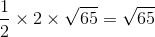Since twice the area of the base is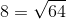, the problem comes down to comparing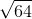and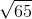; the latter, which is (B), is greater.